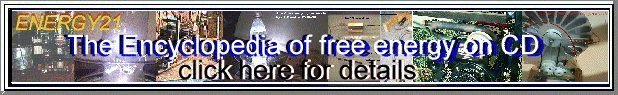### Basic Circuit Theory

Circuit theory for high voltage systems is essentially the same as for any other circuit. Ohm's law and Kirchoff's Voltage and Current laws still apply. The actual circuits for most high voltage equipment are actually quite simple, so not much analytical work is necessary to get an understanding of the expected behavior. In fact, slavish use of mathematical circuit modelling may not be the best approach for high voltage circuits, because the characteristics of the components are not known accurately, and the effects of dielectric breakdown (e.g. corona) are unpredictable. In much of high voltage engineering, empiricism still rules the day.

#### RC circuits

discharge: V(t) = Vinitial * EXP ( -t / (R*C))

The product R*C is referred to as the time constant.

#### Nonlinear elements

The most common non-linear circuit encountered in high voltage circuits is a spark or arc. The voltage drop across a high pressure arc (e.g. in a xenon flash tube) is proportional to the square root of the current. This is known as the Goncz relation.

E = K0 * SQRT(I)

Another common non-linear element is the essentially constant voltage glow discharge, typified by a neon lamp. In fact, glow discharges can have a negative resistance characteristic, in that the voltage drop across the discharge decreases as the current increases.

In some simple cases (like a xenon flash tube discharging in a simple RLC loop), a fairly accurate analytical solution can be determined. In more complex cases, numerical integration is the best approach.

Copyright 1997, Jim Lux / circuit.htm / Back to HV Home / Back to home page / Mail to Jim